Students can Download Maths Chapter 1 Number System Ex 1.3 Questions and Answers, Notes Pdf, Samacheer Kalvi 7th Maths Book Solutions Guide Pdf helps you to revise the complete Tamilnadu State Board New Syllabus and score more marks in your examinations.

## Tamilnadu Samacheer Kalvi 7th Maths Solutions Term 3 Chapter 1 Number System Ex 1.3

Question 1.
Find the product of the following
(i) 0.5 × 3
(ii) 3.75 × 6
(iii) 50.2 × 4
(iv) 0.03 × 9
(v) 453.03 × 7
(vi) 4 × 0.7
Solution:
(i) 0.5 × 3
5 × 3 = 15
∴ 0.5 × 3 = 1.5

(ii) 3.75 × 6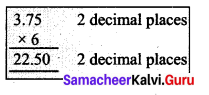375 × 6 = 2250
3.75 × 6 = 22.50(iii) 50.2 × 4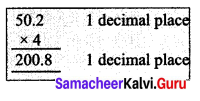502 × 4 = 2008
50.2 × 4 = 200.8

(iv) 0.03 × 9
3 × 9 = 27
0.03 × 9 = 0.27

(v) 453.03 × 7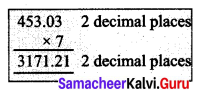45303 × 7 = 317121
453.03 × 7 = 3171.21

(vi) 4 × 0.7
4 × 7 = 28
4 × 0.7 = 2.8

Question 2.
Find the area of the parallelogram whose base is 6.8 cm and height is 3.5 cm.
Solution: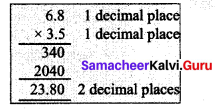Base of the parallelogram b = 6.8 cm
Height of the parallelogram h = 3.5 cm
Area of the parallelogram A = b × h sq.units = 6.8 × 3.5 cm2
Area of the parallelogram = 23.80 cm2Question 3.
Find the area of the rectangle whose length is 23.7 cm and breadth is 15.2 cm.
Solution: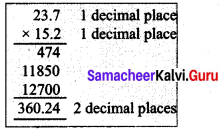Length of the rectangle l = 23.7 cm
Breadth of the rectangle b= 15.2 cm
Area of the rectangle A = l × b sq.units
= 23.7 × 15.2 cm2
Area of the rectangle = 360.24 cm2

Question 4.
Multiply the following

1. 2.57 × 10
2. 0.51 × 10
3. 125.367 × 100
4. 34.51 × 100
5. 62.735 × 100
6. 0.7 × 10
7. 0.03 × 100
8. 0.4 × 1000

Solution:

1. 2.57 × 10 = 25.7
2. 0.51 × 10 = 5.1
3. 125.367 × 100 = 12536.7
4. 34.51 × 100 = 3451
5. 62.735 × 100 = 6273.5
6. 0.7 × 10 = 7.0
7. 0.03 × 100 = 3
8. 0.4 × 1000 = 400Question 5.
A wheel of a baby cycle covers 49.7 cm in one rotation. Find the distance covered in 10 rotations.
Solution:
Length covered in 1 rotation = 49.7 cm
Length covered in 10 rotations = 49.7 × 10 cm = 497 cm

Question 6.
A picture chart costs ₹ 1.50. Radha wants to buy 20 charts to make an album. How much does she have to pay?
Solution: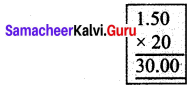Cost of 1 chart = ₹ 1.50
Cost of 20 charts = ₹ 1.50 × 20 = ₹ 30.00
Cost of 20 charts = ₹ 30

Question 7.
Find the product of the following.
(i) 3.6 × 0.3
(ii) 52.3 × 0.1
(iii) 537.4 × 0.2
(iv) 0.6 × 0.06
(v) 62.2 × 0.23
(vi) 1.02 × 0.05
(vii) 10.05 × 1.05
(viii) 101.01 × 0.01
(ix) 100.01 × 1.1
Solution:
(i) 3.6 × 0.3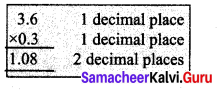36 × 3 = 108
3.6 × 0.3 = 1.08

(ii) 52.3 × 0.1
523 × 1 = 523
52.3 × 0.1 = 5.23

(iii) 537.4 × 0.2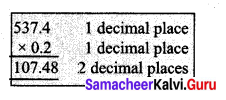5374 × 2 = 10748
537.4 × 0.2 = 107.48(iv) 0.6 × 0.06
6 × 6 = 36
0.6 × 0.06 = 0.036

(v) 62.2 × 0.23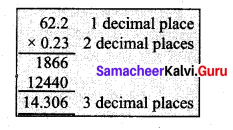622 × 23 = 14306
62.2 × 0.23 = 14.306

(vi) 1.02 × 0.05
102 × 5 = 510
1.02 × 0.05 = 0.0510

(vii) 10.05 × 1.05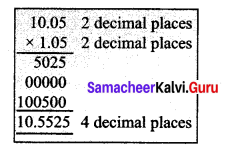1005 × 105 = 105525
10.05 × 1.05 = 10.5525

(viii) 101.01 × 0.01
10101 × 1 = 10101
101.01 × 0.01 = 1.0101

(ix) 100.01 × 1.1
1001 × 11 = 110011
100.01 × 1.1 = 110.011

Objective Type Questions

Question 1.
1.07 × 0.1 _______
(i) 1.070
(ii) 0.107
(iii) 10.70
(iv) 11.07
(ii) 0.107
Hint:
107 × 1 = 107
1.07 × 0.1 = 0.107Question 2.
2.08 × 10 = ______
(i) 20.8
(ii) 208.0
(iii) 0.208
(iv) 280.0
(i) 20.8
Hint:
208 × 10 = 2080
2.08 × 10 = 20.80 = 20.8Question 3.
A frog jumps 5.3 cm in one jump. The distance travelled by the frog in 10 jumps is _______
(i) 0.53 cm
(ii) 530 cm
(iii) 53.0 cm
(iv) 53.5 cm